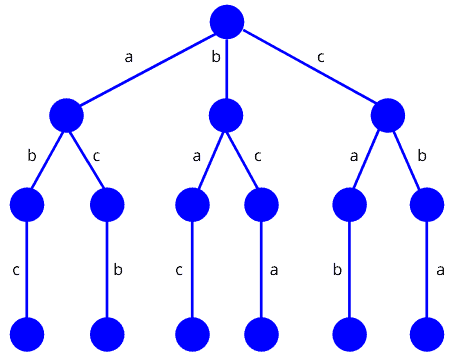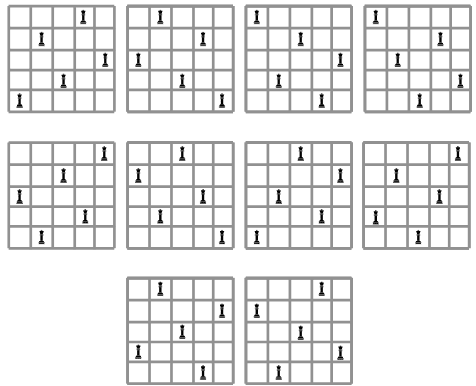## 1. Overview

In this tutorial, we’ll discuss the theoretical idea behind backtracking algorithms. We’ll also present a classic problem that uses the backtracking approach to find a solution.

## 2. Introduction to Backtracking

Backtracking is an algorithmic technique where the goal is to get all solutions to a problem using the brute force approach. It consists of building a set of all the solutions incrementally. Since a problem would have constraints, the solutions that fail to satisfy them will be removed.

It uses recursive calling to find a solution set by building a solution step by step, increasing levels with time. In order to find these solutions, a search tree named state-space tree is used. In a state-space tree, each branch is a variable, and each level represents a solution.

A backtracking algorithm uses the depth-first search method. When it starts exploring the solutions, a bounding function is applied so that the algorithm can check if the so-far built solution satisfies the constraints. If it does, it continues searching. If it doesn’t, the branch would be eliminated, and the algorithm goes back to the level before.

## 3. An Example

We’re taking a very simple example here in order to explain the theory behind a backtracking process. We want to arrange the three lettersin such a way thatcannot be beside.

According to the backtracking, first, we’ll build a state-space tree. We’ll find all the possible solutions and check them with the given constraint. We’ll only keep those solutions that satisfy the given constraint:The possible solutions of the problems would be:,,,,,.

Nevertheless, the valid solutions to this problem would be the ones that satisfy the constraint, which keeps onlyandin the final solution set.

## 4. When to Use a Backtracking Algorithm

The backtracking algorithm is applied to some specific types of problems. For instance, we can use it to find a feasible solution to a decision problem. It was also found to be very effective for optimization problems.

For some cases, a backtracking algorithm is used for the enumeration problem in order to find the set of all feasible solutions for the problem.

On the other hand, backtracking is not considered an optimized technique to solve a problem. It finds its application when the solution needed for a problem is not time-bounded.

## 5. A Backtracking Algorithm

### 5.1. Problem Statement

A classic example of backtracking is the-Queens problem, first proposed by German chess enthusiast Max Bezzel in 1848. Given a chessboard of size, the problem is to placequeens on thechessboard, so no two queens are attacking each other.

It is clear that for this problem, we need to find all the arrangements of the positions of thequeens on the chessboard, but there is a constraint: no queen should be able to attack another queen.

### 5.2. Pseudocode

Let’s see pseudocode that uses backtracking technique to solve-Queens problem:We start this algorithm with an arrayand a parameterthat represents the index of the first empty row. The positions of the queens on achessboard is stored using an array, whereindicates which square in rowcontains a queen.

First, we check if the size of the variableis greater than the size of. If this condition satisfies, we return the array. Ifis less than, we check the queen’s current position with the index value. If it is a non-attacking position for placing a queen on the chessboard, we save the index in the array. Like this, we explore all the positions on the chessboard by calling thefunction recursively.

### 5.3. A Solution Example

If we take achessboard as an example, solving the problem results in 10 solutions, which leads us to use the backtracking algorithm in order to retrieve all these solutions:It is true that for this problem, the solutions found are valid, but still, a backtracking algorithm for the-Queens problem presents a time complexity equal to.

## 6. Conclusion

In this tutorial, we’ve discussed the general idea of the backtracking technique. We also presented an algorithm that uses backtracking.

Backtracking remains a valid and vital tool for solving various kinds of problems, even though this algorithm’s time complexity may be high, as it may need to explore all existing solutions.